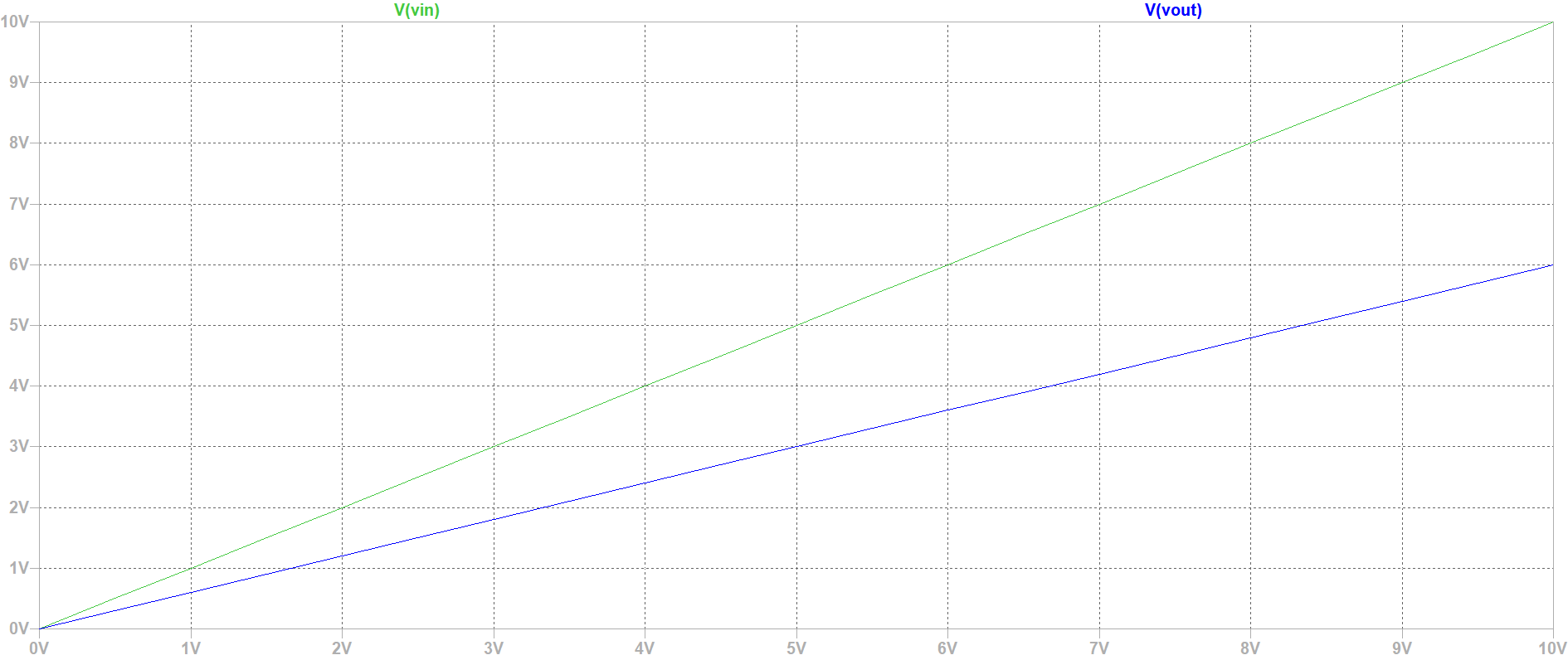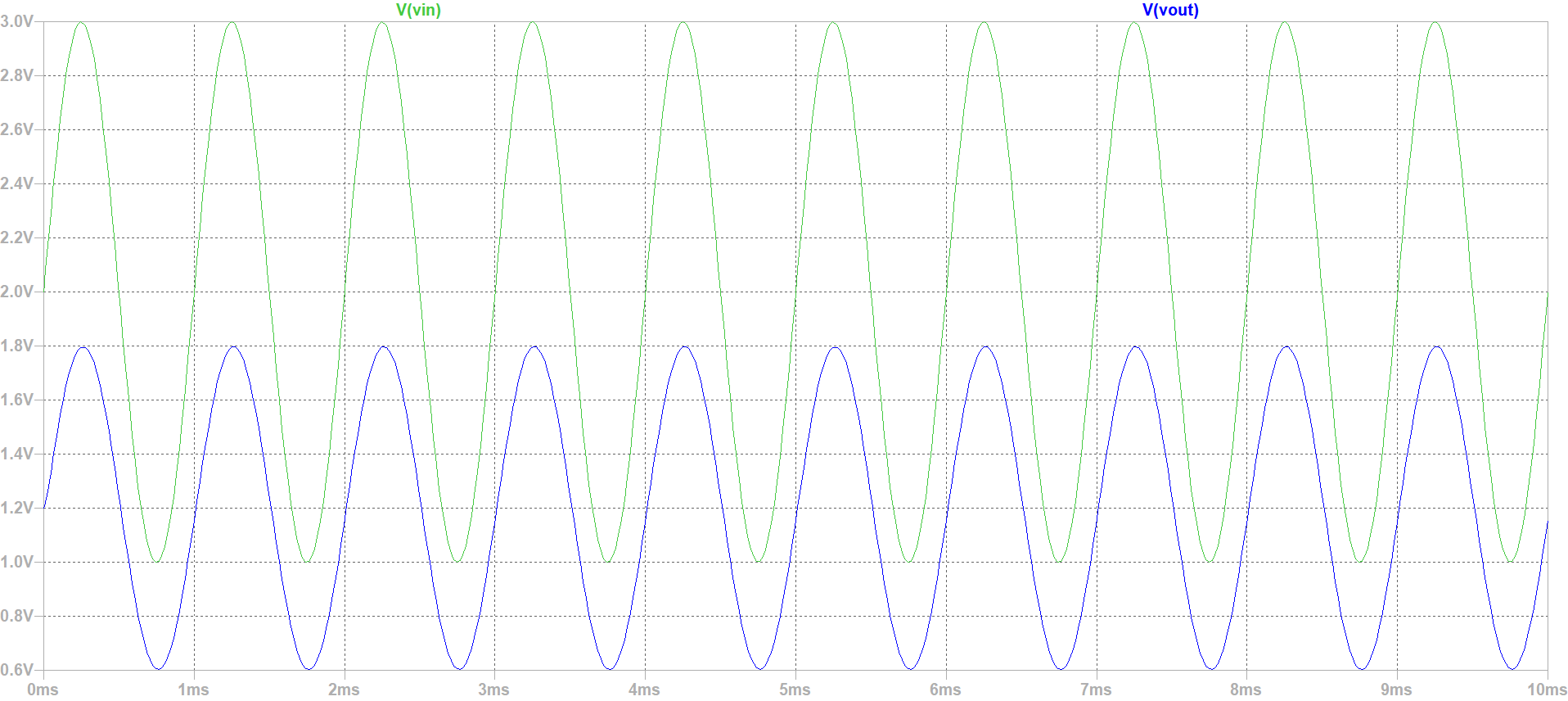# Overview

• LTSPICE configuration and preferences
• RC circuit (Voltage divider) in LTSPICE
• Simulation analysis
• Summary

# Building Voltage Divider in LTSPICE

• starting LTSPICE, doing configuration and preferences
• Building Voltage devider in LTSPICE
• assiging values to the 2 resistors,the capacitior and the voltage
• doing a simulation: starting with DC Operation point simulation and analysing the results
• Doing a second simulation which is DC sweep simulation, and analysing the results
• Changing ou source voltage type to a sinusuidal one, and we change our simulation to a transient one

# DC sweep simulation and analysis

 In this simulation we started from 0v to 10v with a step volt of 0.5V. The results show Vin which rise from 0v to 10v, and Vout according to the ration of 2k to 3k resistors rise from 0v to 6v.# DC sweep simulation and analysis

 In this simulation we started from 0v to 10v with a step volt of 0.5V. The results show Vin which rise from 0v to 10v, and Vout according to the ration of 2k to 3k resistors rise from 0v to 6v.# Summary

 This lab was an introduction to LTSPICE. we have done a parameters configuration in a first time,then we have done multiples simulation on a voltage divider. this Lab was a good opportunity to get an overview on LTSPICE and the different simulations mode that it offers.Furthermore we have learned that Notepad++ can be used to edit the raw html code for the webpage

# References

 Making of a Webreport , Vollrath